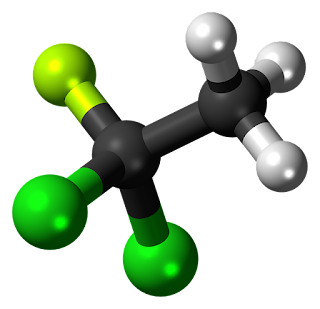# Bond EnergiesWhen two atoms join to form a covalent bond the reaction is exothermic — energy is given out. It therefore follows that to break that covalent bond energy must be supplied. The bond energy is defined as the average standard enthalpy change for the breaking of one mole of bonds in a gaseous molecule to form gaseous atoms:
Br-Br(g) + bond energy → 2Br(g)
Hence bond energy is an indication of the strength of the forces holding the atoms together in a covalent molecule.
Together with bond lengths, bond energies can be used to assess the suitability of a proposed Lewis structure. Bond energy, bond length, and bond order are interrelated properties in this sense: the higher the bond order, the shorter the bond between two atoms and the grexater the bond energy.

Energy is released when isolated atoms join to form a covalent bond, and energy must be absorbed to break apart covalently bonded atoms. Bond dissociation energy, D, is the quantity of energy required to break one mole of covalent bonds in a gaseous species. The SI units are kilojoules per mole of bonds.

It is not hard to picture the meaning of bond energy for a diatomic molecule, because there is only one bond in the molecule. It is also not difficult to see that the bond-dissociation energy of a diatomic molecule can be expressed rather precisely, as is that of H2. With a polyatomic molecule, such as H2O
the situation is different. The energy needed to dissociate one mole of H atoms by breaking one O-H bond per H2O molecule is different from the energy required to dissociate one mole of H atoms by breaking the bonds in OH.

The two O-H bonds in HO are identical; therefore, they should have identical energies. This energy, which we can call the O-H  bond energy in H2O is the average of the two values listed above: 463.4 kj/mole. The O-H bond energy in other molecules containing the OH group will be somewhat different from that in H-O-H. For example, in methanol, the O-H  bond-dissociation energy, which we can represent as D(H-OCH3)is 436.8 kJ per mole. The usual method of tabulating bond energies  is as averages. An average bond energy is the average of bond-dissociation energies for a number of different species containing the particular bond. Understandably, average bond energies cannot be stated as precisely as specific bond-dissociation energies.